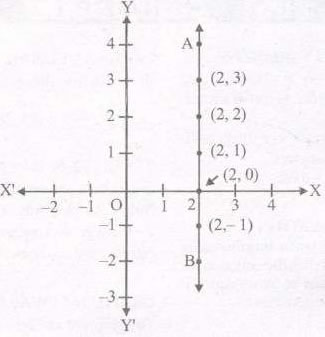# Equations In Two Variables Notes For Class 9 Formulas Download PD

## Linear Equations In Two Variables

### LINEAR EQUATION IN TWO VARIABLES

An equation of the form ax + by + c = 0 where a, b, c are real numbers and x, y are variables, is called a linear equation in two variables.

Here ‘a’ is called coefficient of x, ‘b’ is called coefficients of y and c is called constant term.

Eg. 6x + 2y + 5 = 0, 5x – 2y + 3 = 0 etc.

### SOLUTIONS OF LINEAR EQUATION

The value of the variable which when substituted for the variable in the equation satisfies the equation i.e. L.H.S. and R.H.S. of the equation becomes equal, is called the solution or root of the equation.

### RULES FOR SOLVING AN EQUATION

(i) Same quantity can be added to both sides of an equation without changing the equality.

(ii) Same quantity can be subtracted from both sides of an equation without changing the equality.

(iii) Both sides of an equation may be multiplied by a same non-zero number without changing the equality.

(iv) Both sides of an equation may be divided by a same non-zero number without changing the equality.

### A LINEAR EQUATION EN TWO VARIABLES HAS INFINITELY MANY SOLUTIONS

We can get many many solutions in the following way.

Pick a value of your choice for x (say x = 2) in 2x + 3y = 12. then the equation reduces to 4+3y = 12, which is a linear equation in one variable. On solving this, you get y = 8/3. So (2, 8/3) is another solution of 2x + 3y = 12. Similarly, choosing x = —5, you find that the equation becomes — 10 + 3y = 12. This gives y =22/3. So, (-5, 22/3) is another solution of 2x + 3y = 12. So there is no end to different solutions of a linear equation in two variables.

Note : An easy way of getting a solution is to take x = 0 and get the corresponding value of y. Similarly, we can put y =0 and obtain the corresponding value of x.

### GRAPH OF LINEAR EQUATIONS

The graph of an equation in x and y is the set of all points whose coordinates satisfy the equation :

In order to draw the graph of a linear equation ax + by + c = 0 may follow the following algorithm.

Step 1: Obtain the linear equation ax + by+ c = 0

Step 2 : Express yin terms of x i.e. y = -((ax + b)/c))

Step 3 : Put any two or three values for x and calculate the corresponding values of y from the expression values of y from the expression obtained in step 2. Let we get points as (α1, β1), (α2, β2), (α3, β3)

Step 4 : Plot points (α1, β1), (α2, β2), (α3, β3) on graph paper.

Step 5 : Join the points marked in step 4 to obtain. The line obtained is the graph of the equation ax+by+c= 0

Note : (i) The reason that a degree one polynomial equation ax + by + c = 0 is called a linear equation is that its geometrical representation is a straight line.

(ii) The graph of the equation of the form y = kx is a line which always passes through the origin.

### EQUATIONS OF LINES PARALLEL TO THE X— AXIS AND Y— AXIS

Every point on the x – axis is of the form (x, 0). The equation of the x – axis is given byy 0. Note that y =0 can be expressed as 0. x + 1.)) 0.

Similarly, observe that the equation of they – axis is given by x = 0.

Consider the equation x -2 = 0. If this is treated as an equation in one variable x only, then it has the unique solution x= 2, which is a point on the number line.

An equation in two variables, x -2 = 0 is represented by the line AB in the graph in below given figNote : (i) The graph ofx = a is a straight line parallel to they – axis

(ii) The graph ofy = a is a straight line parallel to thex – axis.

### NCERT Books Free Pdf Download for Class 5, 6, 7, 8, 9, 10 , 11, 12 Hindi and English Medium

 Mathematics Biology Psychology Chemistry English Economics Sociology Hindi Business Studies Geography Science Political Science Statistics Physics Accountancy